Home » All Aboard the Pandas Express – How to Speed up Data Preprocessing using Pandas in Python

# All Aboard the Pandas Express – How to Speed up Data Preprocessing using Pandas in Python

## Overview

• Pandas is the Swiss Army Knife of data preprocessing tasks in Python but can be cumbersome when dealing with large amounts of data
• Learn how to leverage Pandas in Python to become a more efficient data science professional

## Introduction

Pandas is such a popular library that even non-Python programmers and data science professionals have heard plenty about it. And if you’re a seasoned Python programmer, then you’ll be intimately familiar with how flexible the Pandas library is.

But the issue with Pandas is that it can be unbearably slow in certain situations – especially when we’re dealing with huge amounts of data. And in today’s deadline-driven world, efficiency is often what separates successful data science projects from the failed ones.

So how do we go about improving our efficiency in data preprocessing tasks? Can we leverage Pandas, with a few tweaks, and speed up our data science tasks?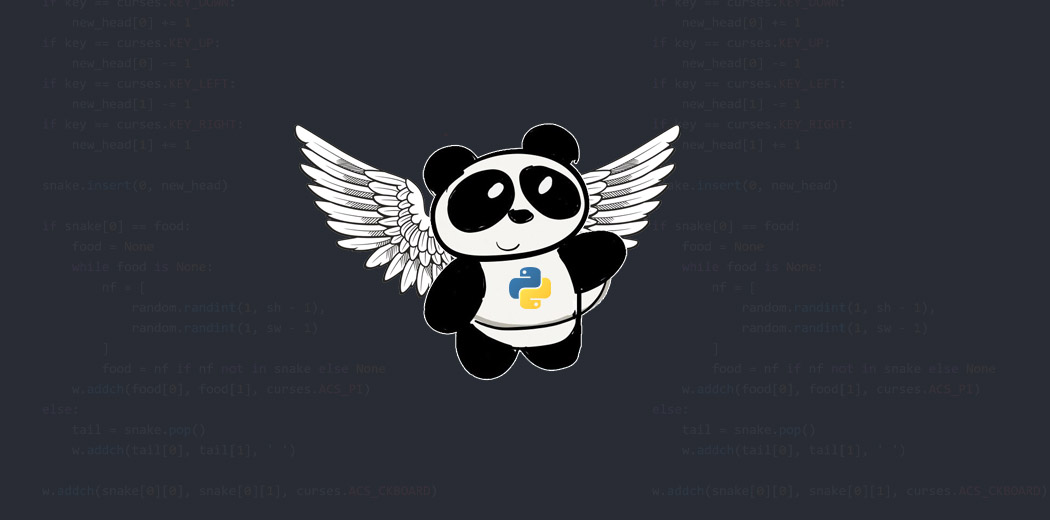In this article, we will focus on two common data preprocessing tasks that I regularly see data scientists struggling with:

1. Binning
2. Adding rows to a dataframe

We’ll talk about different methods to perform these two tasks and find the fastest method that can boost efficiency. And we’ll do all of this with the help of Pandas!

I highly recommend going through the below free courses to become a more efficient data scientist or analyst:

1. Let’s Load the Dataset into our Python Environment
1. Approach 1: Brute-force
2. Approach 2: iterrows()
3. Approach 3: apply()
4. Approach 4: cut()
1. Approach 1: Using the append function
2. Approach 2: Concat function

## Let’s Load the Dataset into our Python Environment

This is going to be as hands-on as possible. I personally believe in learning by doing – and that’s the idea we’ll follow in this article.

We will find the fastest techniques for both these preprocessing tasks and leverage some lesser-known Pandas functions to accomplish this. Now, let us fire up our Jupyter Notebooks or IDEs, and load a dataset.

We will use the ‘cars’ dataset for our experiments. You can either download it from here or if you are using the seaborn library for visualization – it already has the ‘cars’ dataset.

```# Importing Libraries
import pandas as pd
import numpy as np
import matplotlib.pyplot as plt
%matplotlib inline
import seaborn as sns```

Let’s load the dataset into a dataframe:

```mpg = sns.load_dataset('mpg')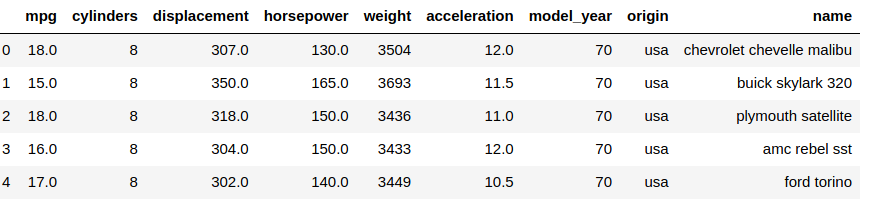For the uninitiated, binning is the conversion of a continuous variable into a categorical variable. Now, if we want to apply conditions on continuous columns, say on the ‘weights’ column, we can create a new categorical column with:

• weight >  1500 and weight < 2500 as ‘Light’
• weight > 2500 and weight < 3500 as ‘Medium’
• weight > 3500 and weight < 4500 as ‘Heavy’
• weight > 4500 as ‘Very heavy’

Let’s observe the various approaches to perform this task and see whether this preprocessing task can be optimized with Pandas.

#### Approach 1: Brute-force

The slowest brute-force method is to iterate through each row and then categorize that row accordingly. For that, we first create a function which checks for the weight value and assigns the category to it:

```def apply_weights(weight):
if 1500 <= weight < 2500:
category = 'Light'
elif 2500 <= weight < 3500:
category = 'Medium'
elif 3500 <= weight < 4500:
category = 'Heavy'
else:
category = 'Very heavy'
return category```

We then take the ‘weight’ column and pass the value of each row to the above function:

```%%timeit
cat_list = []
for i in range(len(mpg)):
wt = mpg.iloc[i]['weight']
cat = apply_weights(wt)
cat_list.append(cat)
mpg['Wt_Categories'] = cat_list```

Let us leverage the timeit utility to compute the time it takes for the above task. For that, we just add %%timeit to the code cell we want to run.

Output:

`mpg.head()`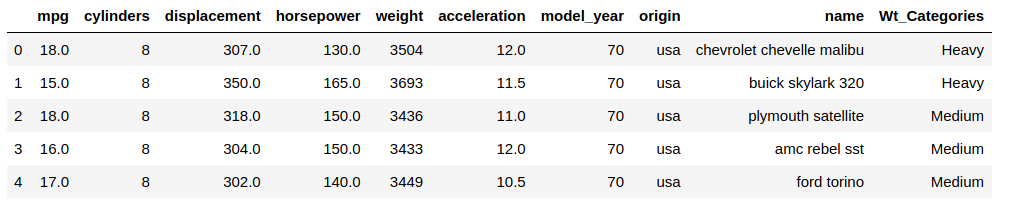And the time it takes for the above task: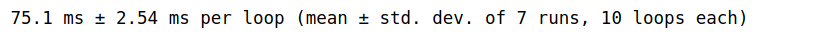Obviously, this loop is taking plenty of time to execute, and the more rows we have, the slower the operation would be. Not ideal!

#### Approach 2: iterrows()

Let’s speed up this process by leveraging the iterrows() function in Pandas. The iterrows function is basically an optimized version of this statement:

`for i in range(len(mpg)):`

This allows us to work with individual rows. As you can see, we have avoided the use of iloc, and the weight column of each row can be accessed just like we do in a dataframe:

```%%timeit
cat_list = []
for index, row in mpg.iterrows():
wt = row['weight']
cat = apply_weights(wt)
cat_list.append(cat)
#print(len(cat_list))
mpg['Wt_Categories_iter'] = cat_list```

Let us confirm the new column:

`mpg.head()`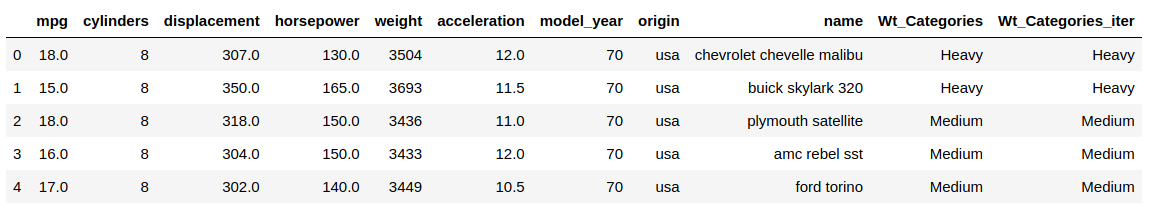and the execution time: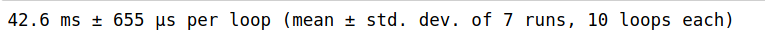Great! We reduced the time taken by a whole 30 ms! Keep in mind that this difference would be even more noticeable for larger datasets and complex functions.

Would you believe me if I say that we can make this much faster? Let us see how.

#### Approach 3: apply()

The apply() method allows applying a given function along a specific axis (0 for rows, and 1 for columns). Internally, apply() also uses for loops, but with a lot of optimizations, which we won’t get into here.

```%%timeit
mpg['wt_cat_apply'] = mpg.apply(lambda row: apply_weights(row['weight']), axis=1)```

Like before, let us confirm the new column and the execution time:

`mpg.head()`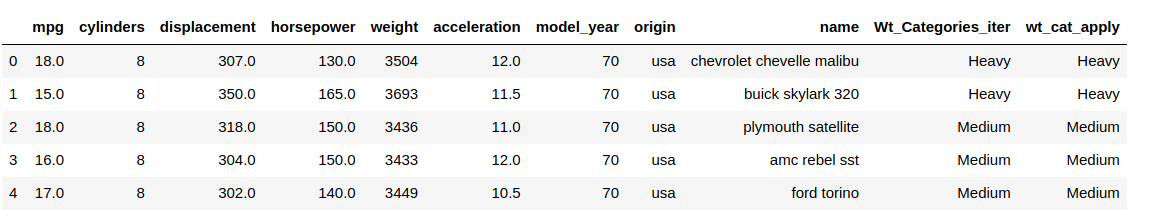and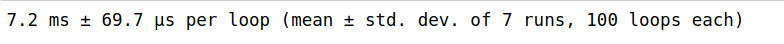Marvelous! Our code started off by taking 75 milliseconds to execute, and we have ended up with the code taking only around 7 milliseconds for the function to execute. Additionally, we were able to accomplish this with a much crisper code as well.

However, if you observe, there were still some constraints in the above methods:

• We still had to write the tedious function apply_weights() and use multiple conditional statements for the weight categories. This might look simple for only 4 categories, but if for 6 or more, we have our work cut out for us
• Also, we cannot always be sure of the lower and upper bounds of each weight category since there can also be a lot of ambiguity in deciding the category intervals
• The category intervals are uneven – this is not intuitive

No sweat – Pandas has a utility to automate the categorization process as well and remove the above constraints!

#### Approach 4: cut()

We use the cut() function of the Pandas library to perform this preprocessing task, and thus, automatically binning our data. We just need to know the four categories we want to bin our column into and the cut function divides the data into these categories.

```%%timeit
mpg['wt_cat_cut'] = pd.cut(np.array(mpg['weight']), 4, labels=["Light", "Medium", "Heavy", "Very heavy"])```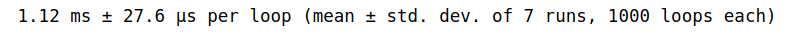And the new column as well: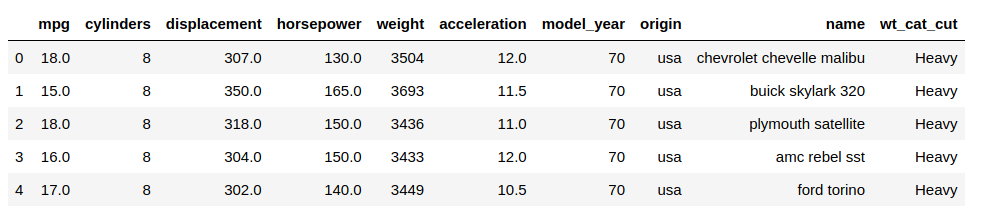Amazing! We took only around 1 ms to categorize our data – a stark difference from the 72 ms we had started out with and a marked improvement over the apply() function.

To summarize, here are our results: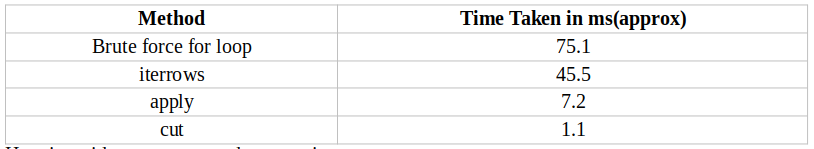Let’s try to add new rows to an existing dataframe. Two common ways to perform this are:

• append
• concat

Both of these belong to the Pandas library and need to be compared to understand which approach helps us in effectively achieving the preprocessing technique of adding rows to a dataframe.

Let us find the fastest way to do so. For this, let us create 3 simple dataframes:

```raw_data = {
'subject_id': ['1', '2', '3', '4', '5'],
'first_name': ['Alex', 'Amy', 'Allen', 'Alice', 'Ayoung'],
'last_name': ['Anderson', 'Ackerman', 'Ali', 'Aoni', 'Atiches']}
df_a = pd.DataFrame(raw_data, columns = ['subject_id', 'first_name', 'last_name'])
df_a```

###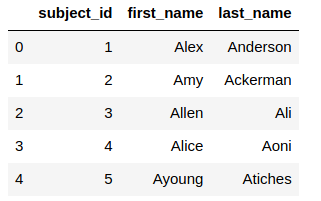``````raw_data = {
'subject_id': ['4', '5', '6', '7', '8'],
'first_name': ['Billy', 'Brian', 'Bran', 'Bryce', 'Betty'],
'last_name': ['Bonder', 'Black', 'Balwner', 'Brice', 'Btisan']}
df_b = pd.DataFrame(raw_data, columns = ['subject_id', 'first_name', 'last_name'])
df_b``````

###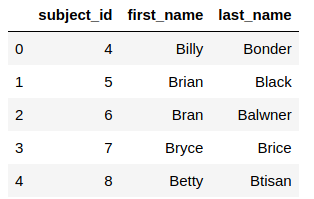```raw_data = {
'subject_id': ['6', '5', '1', '3', '9'],
'first_name': ['Jason', 'Molly', 'Tina', 'Jake', 'Amy'],
'last_name': ['Miller', 'Jacobson', 'Ali', 'Milner', 'Cooze']}
df_c = pd.DataFrame(raw_data, columns = ['subject_id', 'first_name', 'last_name'])
df_c```

###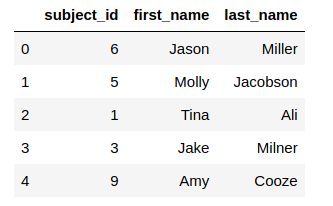#### Approach 1: Using the append function

We will use the append() function to add rows of a dataframe to the end of an existing dataframe. This function is pretty useful since it has parameters like ‘verify_integrity’ which raises an error if rows with duplicate indices are being appended. We can also sort the resulting dataframe by a column using the sort parameter.

While adding rows of a dataframe to another dataframe is pretty simple, it is when we have to add 3 or more dataframes together that we encounter problems. We cannot pass more than one dataframe as an argument to the append function. Thus, we have no choice but to append 2 dataframes and append the result of that to the 3rd dataframe.

This can be particularly tedious when there are more dataframes involved.

Below is the Python code – let us evaluate the time it takes to perform this operation. Please remember to set the ignore_index parameter to True so that the index of the new dataframe ranges from 0 to n-1:

```%%timeit
temp_df = df_a.append(df_b, ignore_index = True)
df_new = temp_df.append(df_c, ignore_index = True)```

What does the new dataframe look like?Let us also look at the time this operation has taken: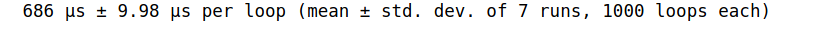That is quite a long time taken for adding 3 dataframes with only 5 rows! Obviously, this would take a much longer time if we are working with larger dataframes.

Can we optimize this and speed up this process as well? Of course, we can. 🙂

#### Approach 2: Concat function

The concat function in Pandas works very similar to the concatenate function in NumPy. One of the advantages of the concat function is the sheer number of parameters it allows, like ‘axis’: 0 or 1 which allows concatenating dataframes by rows or by columns, along with verify_integrity and sort.

The other parameters are used when we want to concatenate by columns. The major advantage over the append function is that we can pass as many dataframes as arguments to the concat function as we want. This results in crisper code as we can add together multiple rows with a single line of code:

```%%timeit
df_concat = pd.concat([df_a, df_b, df_c], axis = 0)```

The resultant dataframe: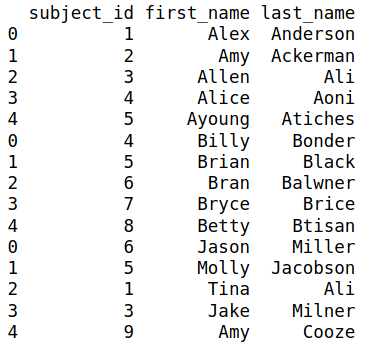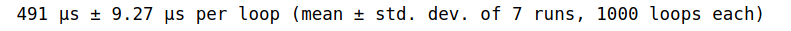Clocking in at around 491 µseconds, it takes much lesser time than the append function. Though this difference might look negligible now, it would be more pronounced for larger dataframes. Additionally, the append() function is internally more inefficient since it involves creating a new object with the merged data.

This being said, if you do want to add individual rows to a dataframe, it is best to use the .loc function:

`df_a.loc = ['5', 'Billy', 'Bounder']`

## End Notes

Here, we observed 2 common preprocessing tasks and compared different approaches to find how the Pandas library effectively and effectively helps us achieve these tasks.

It is important to use the most efficient techniques while preprocessing your data since a data scientist spends most of their time cleaning their data and preparing it for the next steps of data analysis and model building.

Reach out to us if you have any queries in the comments section below. Also, let us know the functions you use or have used to optimize your data preprocessing steps!

You can also read this article on our Mobile APP•furas says:
•furas says:
•furas says: